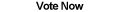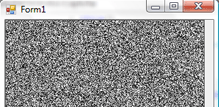Home Articles Components Code Snippets Links# C# Generate Image Noise## Image Noise

Using a simple algorithm, it is possible to generate image noise in C#. Image noise is a collection of pixels of random color. Generating noise in C# could have many possible uses, and while there are some more advanced algorithms, this will be a simple yet useful function.

And in this case the code will generate a grayscale image noise, meaning only the colors white and black and anything in between (grays).

## Black and White Noise

The simplest way to generate image noise with only black and white tones is to randomly pick colors that have equal red, green, and blue values (RGB). Thus for each pixel, the C# function needs to a single random value between 0 and 255 and apply that value for all the RGB values.

In this case we are not going to worry about the alpha value, however, you can image that we could choose a random value between 0 and 255 for the alpha too. Modifying the alpha channel is most useful if you want to draw the image noise over another image.

The randomly generated noise can be constricted to give the overall noise a certain appearance. For example, restricting random values between 0 and 50 yields an overall dark image noise. On the other hand 200 and 255 makes a lighter image noise. The closer the values are to each other the smoother the noise generated will be.

## C# Code

The function is very simple, in fact it uses straight .NET GDI+ calls to make it easy to understand.

```public Bitmap GenerateNoise(int width, int height)
{
Bitmap finalBmp = new Bitmap(width, height);
Random r = new Random();

for (int x = 0; x < width; x++)
{
for (int y = 0; y < height; y++)
{
int num = r.Next(0, 256);
finalBmp.SetPixel(x, y, Color.FromArgb(255, num, num, num));
}
}

return finalBmp;
}```

The function will of course be rather slow, there are ways to make it faster but it makes the code ugly and hard to understand. Also notice that the range values are 0 and 256. The upperbound is exclusive so the values will always be between 0 and 255, which include all the valid values for each RGB value.

As you can tell the C# image noise generating algorithm is very simple, it's nothing more than randomly picking colors. If you want to create image noise that uses all colors, simply get separate random values for each RGB value. Finally for more advanced noise generating algorithms here is a good place to start on advanced image processing in C#.

Back to C# Article List

Other C# Articles